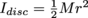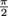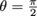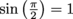Study Guide

# Rotation Questions

## Rotation Questions

1. Why do we often say that angular motion is analogous to translational motion?

Answer: Angular motion is analogous to translational motion because Newton's laws still hold—however, F = ma becomes τ = Iα when we talk about rotation. Quantities that appear in translational motion (position, velocity, acceleration, mass, momentum, and force) all have direct counterparts in rotational motion (angle, angular velocity, angular acceleration, moment of inertia, angular momentum, and torque). The formulas that describe both motions are largely interchangeable with these substitutions.

2. How do you find the tangential speed of a point with a given angular velocity? What about the angular velocity of a point with a given tangential velocity?

Answer: To find a rotating point's tangential velocity, multiply by the radius of the circle the point is moving in. To find the angular velocity of a moving object, divide its translational speed by the radius of the circle it's moving in.

3. Why do points on the inside of a record move slower than points on the outside?

Answer: The entire record rotates with the same angular velocity. Because of this, points on the inside of the record must have a lower angular velocity—they travel around in a smaller circle, but make the same number of revolutions per minute as points on the outside of the record, which move in a much larger circle.

4. What is the necessary condition for an object to travel in a perfect circle?

Answer: In order for an object to move in a perfect circle, the sum of all forces acting on it must point towards the center of the circle. This keeps the object's speed constant, but changes the direction of its motion, resulting in a circular trajectory. When the sum of all forces on an object points towards the middle of a circle, we call this sum the centripetal force felt by the rotating object. Centripetal force is not a force in its own right—only the sum of other forces that happens to be pointing in the right direction.

5. Does a heavier object always have a higher moment of inertia than a lighter object?

Answer: No. Moment of inertia is more dependent on the shape of an object than its mass—a hoop has twice the moment of inertia of a similarly sized disc, for example (Ihoop = Mr2 vs.), so the hoop can weigh half as much as the disc and still have the same moment of inertia.

6. Can an object move if all the forces on it sum to zero?

Answer: Yes—it can't translate if all the forces are balanced, but it can rotate. This situation is called a couple or a moment, and happens when equal and opposite forces are applied on opposite sides of the pivot of a rotating object. The forces cancel each other out, but both forces create a torque in the same direction—so the two torques sum, rather than cancel. Newton's Second Law for rotation (τ = Iα) applies.

7. What quantities are conserved in an inelastic collision of two rolling objects? In an elastic collision?

Answer: Both linear momentum and angular momentum are conserved in both cases—conserved separately, however: the total amount of linear momentum does not change, and neither does the total amount of angular momentum. Kinetic energy is not conserved in an inelastic collision (some energy is lost to heat, sound, noise, etc.). In an elastic collision, overall kinetic energy is conserved—though some of it may move from translational kinetic energy to rotational kinetic energy or vice versa.

8. What angle between a force and its lever arm maximizes the torque that the force can produce?

Answer: Torque is maximized when the angle between a force and the lever arm it acts over is. This is seen in the equation for torque: τ = rF sin θ, where the maximum value of sin θ is achieved at: that is,. At a right angle, the most force can be used to directly rotate an object.

9. Why does a banked curve help race cars turn?

Answer: Banking a curve tilts the normal force that the road exerts on the car. Instead of friction from the tires on the asphalt being the only force that contributes to the centripetal force and keeps the car moving in a circle, the horizontal component of this tilted normal force also contributes when a turn is banked. A larger centripetal force—one that is the sum of a frictional component and normal component—means the car can stay on a tighter circular path (smaller r) with a higher speed (v).

10. Why is it harder to open a door by pushing near the jamb than the handle?

Answer: The further away from the door hinges you push on the door—that is, by the handle—the more torque you can create with the same amount of force. It is difficult to create enough torque to turn open a door (especially a heavy door) by pushing near the jamb, which gives you a very small lever arm.